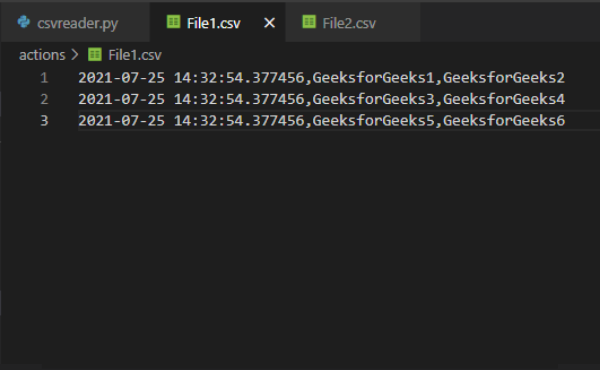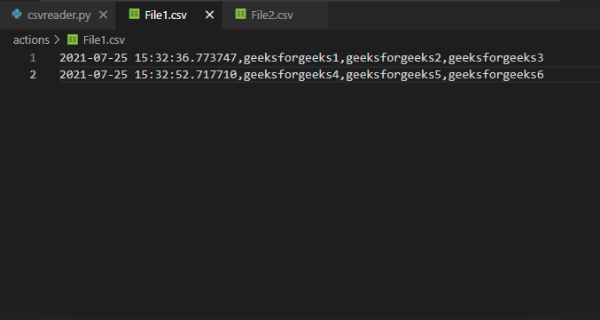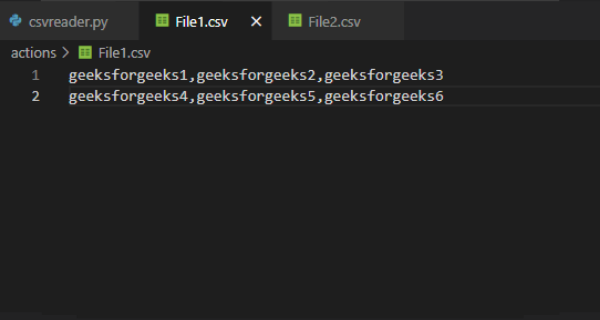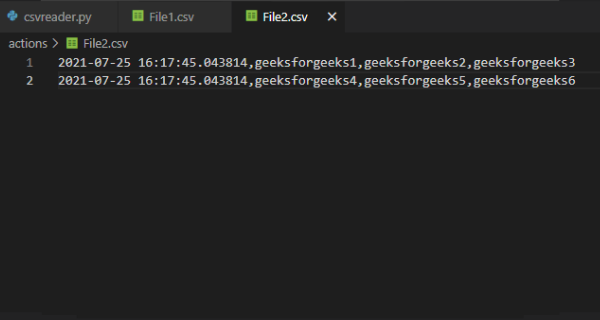# How to add timestamp to CSV file in Python

Prerequisite: Datetime module

In this example, we will learn How to add timestamp to CSV files in Python. We can easily add timestamp to CSV files with the help of datetime module of python. Let’s the stepwise implementation for adding timestamp to CSV files in Python.

## Creating CSV and adding timestamp

• Import csv and datetime module. We will use the csv module to read and write the csv file and datetime module to add the current date and time in the csv file
• Take the data from the user.
• Open the CSV file in read and write mode (‘r+’) using open() function.
• The open() function opens a file and returns its as a file-object.
• newline = ‘ ‘ controls how universal newlines mode works. It can be None, ‘ ‘, ‘\n’, ‘\r’, and ‘\r\n’.
• write() returns a writer object which is responsible for converting the user’s data into a delimited string.
• Get current date and time using the datetime.now() function of datetime module.
• Iterate over all the data in the rows variable with the help of a for loop.
• Insert the current date and time at 0th index in every data using the insert() function.
• Write the data using writerow() in the CSV file with the current date and time.

Example 1: Add timestamp to CSV file

## Python3

 `# Importing required modules ` `import` `csv ` `from` `datetime ``import` `datetime ` ` `  ` `  `# Here we are storing our data in a ` `# variable. We'll add this data in ` `# our csv file ` `rows ``=` `[[``'GeeksforGeeks1'``, ``'GeeksforGeeks2'``], ` `        ``[``'GeeksforGeeks3'``, ``'GeeksforGeeks4'``], ` `        ``[``'GeeksforGeeks5'``, ``'GeeksforGeeks6'``]] ` ` `  `# Opening the CSV file in read and ` `# write mode using the open() module ` `with ``open``(r``'YOUR_CSV_FILE.csv'``, ``'r+'``, newline``=``'') as ``file``: ` ` `  `    ``# creating the csv writer ` `    ``file_write ``=` `csv.writer(``file``) ` ` `  `    ``# storing current date and time ` `    ``current_date_time ``=` `datetime.now() ` ` `  `    ``# Iterating over all the data in the rows  ` `    ``# variable ` `    ``for` `val ``in` `rows: ` `         `  `        ``# Inserting the date and time at 0th  ` `        ``# index ` `        ``val.insert(``0``, current_date_time) ` `         `  `        ``# writing the data in csv file ` `        ``file_write.writerow(val) `

Output :Example  2: Adding timestamp to CSV file

## Python3

 `# Importing required modules ` `import` `csv ` `from` `datetime ``import` `datetime ` ` `  ` `  `# function to write in csv file ` `def` `write_in_csv(rows): ` ` `  `    ``# Opening the CSV file in read and ` `    ``# write mode using the open() module ` `    ``with ``open``(r``'YOUR_CSV_FILE.csv'``, ``'r+'``, newline``=``'') as ``file``: ` ` `  `        ``# creating the csv writer ` `        ``file_write ``=` `csv.writer(``file``) ` ` `  `        ``# Iterating over all the data in the rows  ` `        ``# variable ` `        ``for` `val ``in` `rows: ` `           `  `            ``# writing the data in csv file ` `            ``file_write.writerow(val) ` ` `  ` `  `# list to store the values of the rows ` `rows ``=` `[] ` ` `  `# while loop to take ` `# inputs from the user ` `run ``=` `'' ` `while` `run !``=` `'no'``: ` ` `  `    ``# lists to store the user data ` `    ``val ``=` `[] ` ` `  `    ``# Taking inputs from the user ` `    ``val1 ``=` `input``(``"Enter 1st value:- "``) ` `    ``val2 ``=` `input``(``"Enter 2nd value:- "``) ` `    ``val3 ``=` `input``(``"Enter 3rd value:- "``) ` ` `  `    ``# storing current date and time ` `    ``current_date_time ``=` `datetime.now() ` ` `  `    ``# Appending the inputs in a list ` `    ``val.append(current_date_time) ` `    ``val.append(val1) ` `    ``val.append(val2) ` `    ``val.append(val3) ` ` `  `    ``# Taking input to add one more row ` `    ``# If user enters 'no' then the will loop will break ` `    ``run ``=` `input``(``"Do you want to add one more row? Type Yes or No:- "``) ` `    ``run ``=` `run.lower() ` ` `  `    ``# Adding the stored data in rows list ` `    ``rows.append(val) ` ` `  `# Calling function to write in csv file ` `write_in_csv(rows) `

Output:## Adding Timestamps in Existing CSV file

It is also possible to add timestamp to a CSV file that already contains some data. For this open the first file in read mode and the second file in write mode. Creating a csv reader object of the first file using the reader() function of csv module. reader() return a reader object which will iterate over lines in the given CSV file.

Append every data stored in the first file in rows variable using a for loop. Create a writer object of the second file using the writer() function of csv module. Now iterate over all the data in the rows variable using a for loop. Store the current date and time in a variable and then inserting it in the data at 0th index using the insert() function. Write the stored data in File2 using the writerow() function of csv module.

Example 1: Adding timestamp to existing data

Content of File1:## Python3

 `# Importing required modules ` `import` `csv ` `from` `datetime ``import` `datetime ` ` `  ` `  `# creating a list to store the ` `# existing data of CSV file ` `rows ``=` `[] ` ` `  `# Opening the CSV file in read mode using  ` `# the open() module ` `with ``open``(r``'FILE1.csv'``, ``'r'``, newline``=``'') as ``file``: ` `     `  `    ``# Opening another CSV file to in write mode  ` `    ``# to add the data ` `    ``with ``open``(r``'FILE2.csv'``, ``'w'``, newline``=``'') as file2: ` ` `  `        ``# creating the csv reader ` `        ``reader ``=` `csv.reader(``file``, delimiter``=``','``) ` ` `  `        ``# storing the data of the csv file in a list ` `        ``for` `row ``in` `reader: ` `            ``rows.append(row) ` ` `  `         ``# creating the csv writer ` `        ``file_write ``=` `csv.writer(file2) ` ` `  `        ``# Iterating over all the data in the rows ` `        ``# variable ` `        ``for` `val ``in` `rows: ` ` `  `            ``# storing current date and time in a ` `            ``# variable ` `            ``current_date_time ``=` `datetime.now() ` `            ``val.insert(``0``, current_date_time) ` `             `  `            ``# writing the data in csv file ` `            ``file_write.writerow(val) `

Output:Whether you're preparing for your first job interview or aiming to upskill in this ever-evolving tech landscape, GeeksforGeeks Courses are your key to success. We provide top-quality content at affordable prices, all geared towards accelerating your growth in a time-bound manner. Join the millions we've already empowered, and we're here to do the same for you. Don't miss out - check it out now!

Previous
Next